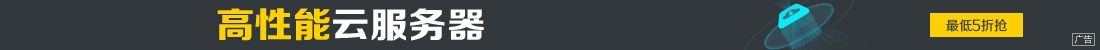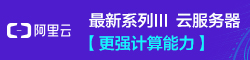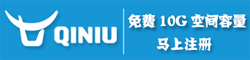# PHP迭代器和生成器用法实例分析

••```<?php
class Sample implements Iterator{
private \$curIndex=0;
private \$items=null;
public function __construct(\$_items) {
\$this->items = \$_items;
}
public function current (){
echo "current\n";
return \$this->items[\$this->curIndex];
}
public function key (){
echo "key\n";
return \$this->curIndex;
}
public function next (){
echo "next\n";
\$this->curIndex++;
}
public function rewind (){
\$this->curIndex = 0;
}
public function send ( \$value ){
if(\$value == "stop"){
\$this->curIndex = null;
}
}
public function valid (){
echo "valid\n";
return isset(\$this->items[\$this->curIndex]);
}
}
\$sample = new Sample([1,2,3]);
foreach (\$sample as \$k =>\$v){
}

```

valid current key next

PHP 将会在每次需要值的时候调用生成器函数，并在产生一个值之后保存生成器的状态，这样它就可以在需要产生下一个值的时候恢复调用状态。

```<?php
function gen_one_to_three() {
for (\$i = 1; \$i <= 3; \$i++) {
//注意变量\$i的值在不同的yield之间是保持传递的。
yield \$i;
}
}
\$generator = gen_one_to_three();
foreach (\$generator as \$value) {
echo "\$value\n";
}
var_dump(\$generator); //实际上是Generator对象

```

```function myYeild(){
\$args = func_get_args();
return new Sample(\$args);
}
\$generator = myYeild(1,2,3);
foreach (\$generator as \$value) {
echo "\$value\n";
}

```

• 数据库遍历

```class AllUser implements \Iterator
{
protected \$index = 0;
protected \$data = [];
public function __construct()
{
\$link = mysqli_connect('192.168.0.91', 'root', '123', 'xxx');
\$this->data = mysqli_fetch_all(\$rec, MYSQLI_ASSOC);
}
//1 重置迭代器
public function rewind()
{
\$this->index = 0;
}
//2 验证迭代器是否有数据
public function valid()
{
return \$this->index < count(\$this->data);
}
//3 获取当前内容
public function current()
{
\$id = \$this->data[\$this->index];
return User::find(\$id);
}
//4 移动key到下一个
public function next()
{
return \$this->index++;
}
//5 迭代器位置key
public function key()
{
return \$this->index;
}
}
//实现迭代遍历用户表
\$users = new AllUser();
//可实时修改
foreach (\$users as \$user){
\$user->save();
}

```
• 文件遍历
一次读取一行
• 实现Iterator接口，让普通类可以使用foreach遍历。
• 协程，参见鸟哥则这篇文章